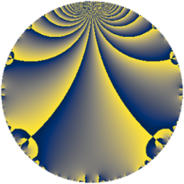# Properties

 Label 7448.2.a.xLevel $7448$ Weight $2$ Character orbit 7448.a Self dual yes Analytic conductor $59.473$ Analytic rank $1$ Dimension $2$ CM no Inner twists $1$

# Related objects

## Newspace parameters

 Level: $$N$$ $$=$$ $$7448 = 2^{3} \cdot 7^{2} \cdot 19$$ Weight: $$k$$ $$=$$ $$2$$ Character orbit: $$[\chi]$$ $$=$$ 7448.a (trivial)

## Newform invariants

 Self dual: yes Analytic conductor: $$59.4725794254$$ Analytic rank: $$1$$ Dimension: $$2$$ Coefficient field: $$\Q(\sqrt{2})$$ Defining polynomial: $$x^{2} - 2$$ Coefficient ring: $$\Z[a_1, a_2, a_3]$$ Coefficient ring index: $$1$$ Twist minimal: no (minimal twist has level 1064) Fricke sign: $$1$$ Sato-Tate group: $\mathrm{SU}(2)$

## $q$-expansion

Coefficients of the $$q$$-expansion are expressed in terms of $$\beta = \sqrt{2}$$. We also show the integral $$q$$-expansion of the trace form.

 $$f(q)$$ $$=$$ $$q + ( -2 + \beta ) q^{3} + q^{5} + ( 3 - 4 \beta ) q^{9} +O(q^{10})$$ $$q + ( -2 + \beta ) q^{3} + q^{5} + ( 3 - 4 \beta ) q^{9} + ( 1 + 3 \beta ) q^{11} + ( 2 - \beta ) q^{13} + ( -2 + \beta ) q^{15} + ( 4 - 2 \beta ) q^{17} + q^{19} + ( 3 - \beta ) q^{23} -4 q^{25} + ( -8 + 8 \beta ) q^{27} + ( -8 + \beta ) q^{29} + ( -6 - \beta ) q^{31} + ( 4 - 5 \beta ) q^{33} + ( -8 - \beta ) q^{37} + ( -6 + 4 \beta ) q^{39} -4 \beta q^{41} + ( 1 + 3 \beta ) q^{43} + ( 3 - 4 \beta ) q^{45} + ( -3 - 3 \beta ) q^{47} + ( -12 + 8 \beta ) q^{51} + ( -4 + \beta ) q^{53} + ( 1 + 3 \beta ) q^{55} + ( -2 + \beta ) q^{57} + ( -2 - 2 \beta ) q^{59} + 5 q^{61} + ( 2 - \beta ) q^{65} + ( 6 + 2 \beta ) q^{67} + ( -8 + 5 \beta ) q^{69} + ( -12 - 3 \beta ) q^{71} + 7 q^{73} + ( 8 - 4 \beta ) q^{75} + ( -6 - 7 \beta ) q^{79} + ( 23 - 12 \beta ) q^{81} + ( -5 + 7 \beta ) q^{83} + ( 4 - 2 \beta ) q^{85} + ( 18 - 10 \beta ) q^{87} + ( 2 + 11 \beta ) q^{89} + ( 10 - 4 \beta ) q^{93} + q^{95} -4 \beta q^{97} + ( -21 + 5 \beta ) q^{99} +O(q^{100})$$ $$\operatorname{Tr}(f)(q)$$ $$=$$ $$2q - 4q^{3} + 2q^{5} + 6q^{9} + O(q^{10})$$ $$2q - 4q^{3} + 2q^{5} + 6q^{9} + 2q^{11} + 4q^{13} - 4q^{15} + 8q^{17} + 2q^{19} + 6q^{23} - 8q^{25} - 16q^{27} - 16q^{29} - 12q^{31} + 8q^{33} - 16q^{37} - 12q^{39} + 2q^{43} + 6q^{45} - 6q^{47} - 24q^{51} - 8q^{53} + 2q^{55} - 4q^{57} - 4q^{59} + 10q^{61} + 4q^{65} + 12q^{67} - 16q^{69} - 24q^{71} + 14q^{73} + 16q^{75} - 12q^{79} + 46q^{81} - 10q^{83} + 8q^{85} + 36q^{87} + 4q^{89} + 20q^{93} + 2q^{95} - 42q^{99} + O(q^{100})$$

## Embeddings

For each embedding $$\iota_m$$ of the coefficient field, the values $$\iota_m(a_n)$$ are shown below.

For more information on an embedded modular form you can click on its label.

Label $$\iota_m(\nu)$$ $$a_{2}$$ $$a_{3}$$ $$a_{4}$$ $$a_{5}$$ $$a_{6}$$ $$a_{7}$$ $$a_{8}$$ $$a_{9}$$ $$a_{10}$$
1.1
 −1.41421 1.41421
0 −3.41421 0 1.00000 0 0 0 8.65685 0
1.2 0 −0.585786 0 1.00000 0 0 0 −2.65685 0
 $$n$$: e.g. 2-40 or 990-1000 Significant digits: Format: Complex embeddings Normalized embeddings Satake parameters Satake angles

## Atkin-Lehner signs

$$p$$ Sign
$$2$$ $$1$$
$$7$$ $$-1$$
$$19$$ $$-1$$

## Inner twists

This newform does not admit any (nontrivial) inner twists.

## Twists

By twisting character orbit
Char Parity Ord Mult Type Twist Min Dim
1.a even 1 1 trivial 7448.2.a.x 2
7.b odd 2 1 7448.2.a.bd 2
7.d odd 6 2 1064.2.q.k 4

By twisted newform orbit
Twist Min Dim Char Parity Ord Mult Type
1064.2.q.k 4 7.d odd 6 2
7448.2.a.x 2 1.a even 1 1 trivial
7448.2.a.bd 2 7.b odd 2 1

## Hecke kernels

This newform subspace can be constructed as the intersection of the kernels of the following linear operators acting on $$S_{2}^{\mathrm{new}}(\Gamma_0(7448))$$:

 $$T_{3}^{2} + 4 T_{3} + 2$$ $$T_{5} - 1$$ $$T_{11}^{2} - 2 T_{11} - 17$$ $$T_{13}^{2} - 4 T_{13} + 2$$ $$T_{17}^{2} - 8 T_{17} + 8$$

## Hecke characteristic polynomials

$p$ $F_p(T)$
$2$ $$T^{2}$$
$3$ $$2 + 4 T + T^{2}$$
$5$ $$( -1 + T )^{2}$$
$7$ $$T^{2}$$
$11$ $$-17 - 2 T + T^{2}$$
$13$ $$2 - 4 T + T^{2}$$
$17$ $$8 - 8 T + T^{2}$$
$19$ $$( -1 + T )^{2}$$
$23$ $$7 - 6 T + T^{2}$$
$29$ $$62 + 16 T + T^{2}$$
$31$ $$34 + 12 T + T^{2}$$
$37$ $$62 + 16 T + T^{2}$$
$41$ $$-32 + T^{2}$$
$43$ $$-17 - 2 T + T^{2}$$
$47$ $$-9 + 6 T + T^{2}$$
$53$ $$14 + 8 T + T^{2}$$
$59$ $$-4 + 4 T + T^{2}$$
$61$ $$( -5 + T )^{2}$$
$67$ $$28 - 12 T + T^{2}$$
$71$ $$126 + 24 T + T^{2}$$
$73$ $$( -7 + T )^{2}$$
$79$ $$-62 + 12 T + T^{2}$$
$83$ $$-73 + 10 T + T^{2}$$
$89$ $$-238 - 4 T + T^{2}$$
$97$ $$-32 + T^{2}$$